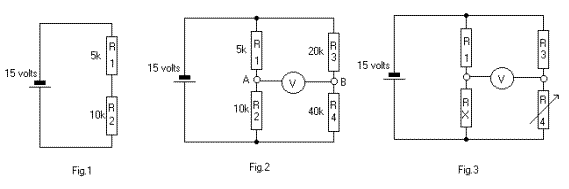Home > Electronics Tutorials > Direct Current (DC) Theory > Wheatstone Bridge Tutorial

Direct Current (DC) Theory - Electronics Teaching

# Wheatstone Bridge Tutorial

Read the page on potential dividers.

In Fig.1, the 15 volts will be divided across the two resistors, according their proportion of the total resistance, 15k.
For the 5k this will be (5k/15k) x 15volts = 5volts.
For the 10k it will be (10k/15k) x 15 volts = 10 volts

In Fig.2 we have the same potential divider plus R3 and R4 across the battery. This is a BRIDGE circuit , invented by Mr. Wheatstone.Using the same calculations as for R1 and R2, we find that the voltage across R3 = 5 volts and across R4 = 10 volts. The voltage has been divided in the same proportions. This is because the ratio R1/R2 is the same as the ratio R3/R4, that is, 1:2. The meter, connected between points A and B will indicate zero. This is because the voltage at both terminals of the meter is the same, so the voltage across the meter is zero. The bridge is said to be BALANCED. So we can say that when the ratio R1/R2 = R3/R4, the bridge is balanced. If the two ratios are not the same, then the voltages at the two terminals of the meter will be different. The meter will now give a reading, and we can say that the bridge is unbalanced.

In Fig.3, Rx is of unknown value and the probability is that the bridge is unbalanced, indicated by a reading on the meter.

If we adjust R4 so that the ratio R1/Rx = R3/R4 then the bridge will be balanced.
If we transpose this equation we can get Rx = (R4 xR1)/R3
We know the values of R1 and R3.
If we measure the value of R4 we can calculate the unknown Rx.

R4 can be fitted with a pointer and a calibrated scale to give a direct reading of Rx without the need for calculations.

This is the basis of more complex bridge circuits.

Note: To report broken links or to submit your projects, tutorials please email to Webmaster

 Discover